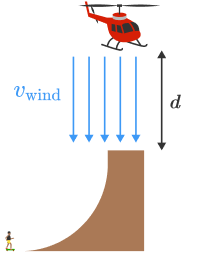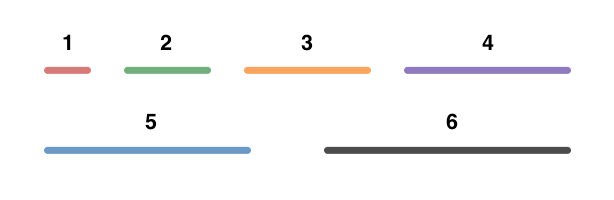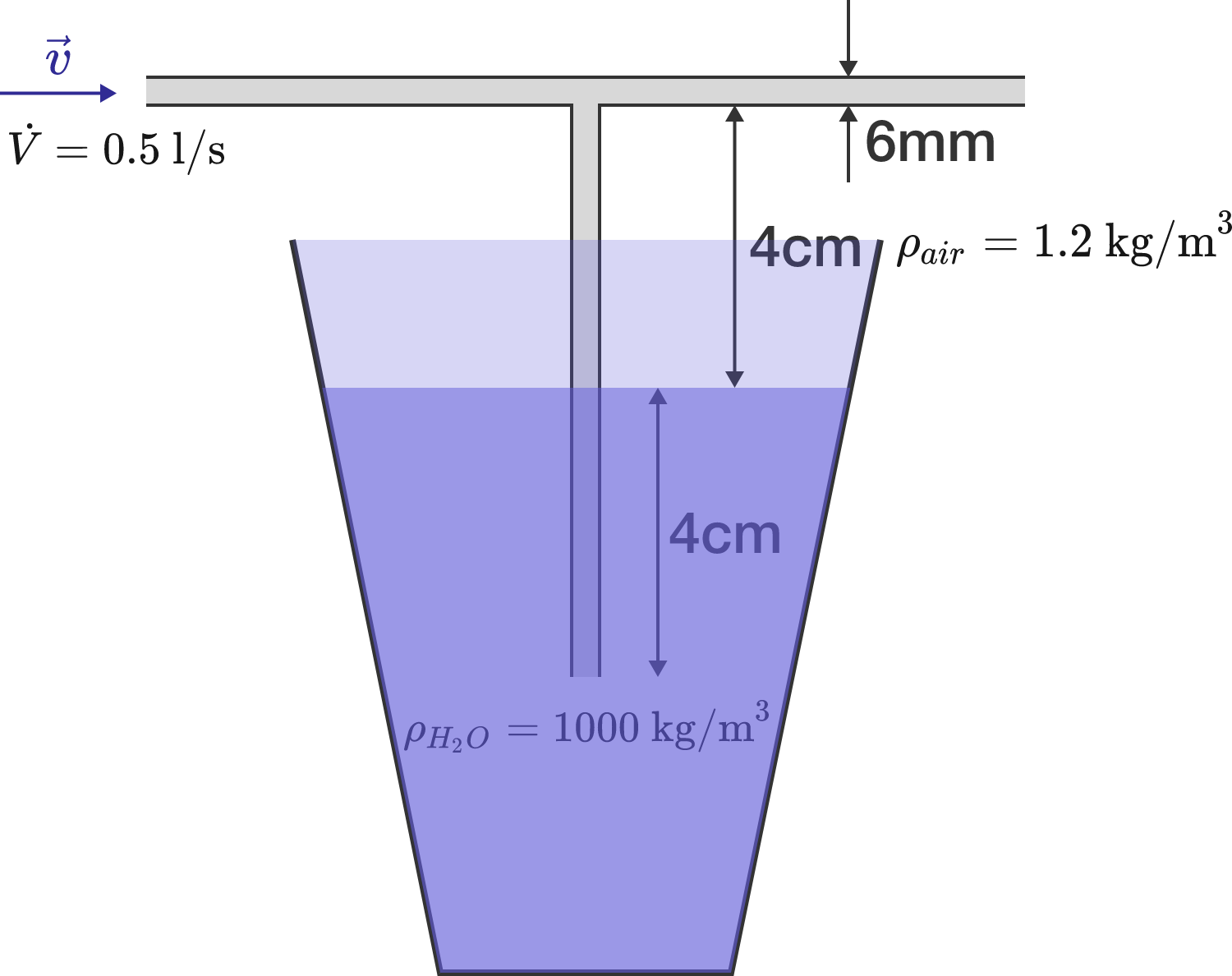# Problems of the Week

Contribute a problem

# 2017-10-02 Intermediate

$\Large \begin{array} { ccccccc} \square \times \square = \square \\ \square + \square = \square \\ \square - \square = \square \\ \end{array}$

Can we fill in each of the squares with a distinct digit such that all of the three equations are true?

$\large \sqrt{8x + 13} + \sqrt{2x - 3} = 5$ Jason came across the above problem in his algebra test and solved it as follows: \begin{aligned} \sqrt{8x + 13} + \sqrt{2x - 3} & = 5 \\ \big(\sqrt{8x + 13} + \sqrt{2x - 3}\big)^2 & = 5^2 \\ 8x + 13 + 2x - 3 & = 25 \\ 10x + 10 & = 25 \\ \Rightarrow x & = \frac 32. \end{aligned} We can see that the cancellation of square roots in step 3 is fallacious. So, what's the correct answer (to 2 decimal places)?

Skateboarder Bob Burnquist has big ramps in his backyard that he's always trying to do bigger and better things with. Recently, Bob had a helicopter pilot hover over his quarterpipe so that he could air and stall on the helicopter's skids.Due to gravity alone Bob has to go pretty fast to air out of the quarterpipe at all but the helicopter provides a massive downward thrust that requires Bob to go even faster. This is a terrifying experience because as you approach the ramp you feel like you have enough speed to air through the blades.

The crucial factor that prevents this is that the air being pushed through the blades works to decelerate Bob's ascent. We can model the drag force on Bob as $\mathbf{F}_\textrm{d} = \frac12 \rho c_\textrm{d} A v_\textrm{relative}^2,$ where $v_\textrm{relative} = v_\textrm{wind} + v_\textrm{Bob}.$

Find Bob's speed at the top of the ramp (in $\si[per-mode=symbol]{\meter\per\second}$) such that he comes to rest exactly at the height of the skids, $d=\SI{4}{\meter}$ above the ramp.

You may want to use the code environment below.

Assume: $v_\textrm{wind} = \SI[per-mode=symbol]{30}{\meter\per\second}$ is the steady windspeed of the air coming down through the blades, Bob's cross sectional area is $A = \SI{1.32}{\meter\squared},$ the drag coefficient for the human body is $c_\textrm{d} = 1,$ $\rho=\SI[per-mode=symbol]{1.22}{\kilo\gram\per\meter\cubed},$ $g=\SI[per-mode=symbol]{10}{\meter\per\second\squared},$ and $m_\textrm{Bob} = \SI{90}{\kilo\gram}.$

import matplotlib.pyplot as plt

g = 10
v_wind = 30
m_bob = 90
C = 0.5 * 1.22 * 1.32

time = 0
height = 0
velocity = 1 # <- You'll want to experiment with this initial value.

(times, heights) = ([], []) # <- Arrays to collect the values of height and time.

# Write code to propagate height and velocity forward in time and
# collect them in the lists, times and heights.

# Uncomment this code to plot h as a function of time.
# plt.plot(times, heights)
# plt.title('$h_\mathrm{max}$ is %f m' % heights[-1])
# plt.xlabel('Time ($t$)')
# plt.ylabel('$h_\mathrm{max}$')
# plt.savefig("my_plot.png")
Python 3
You need to be connected to run code

Does there exist an equiangular (interior angles are equal) hexagon with side lengths $1, 2, 3, 4, 5,$ and $6$?

If yes, is there more than one distinct way (up to rotation and reflection)?As shown in the diagram, a T-shaped straw is dipped into a glass of water. If you blow air through the opening on the left, leaving the opening on the right open, what will happen?

Details and Assumptions:

• The incoming air flow is $\dot V = 0.5\, \text{l/s}.$
• The straw with diameter $6 \,\text{mm}$ has a length of $8 \,\text{cm},$ half of which is immersed in the water.
• The densities of air and water are $\rho = 1.2 \,\text{kg}/\text{m}^3$ and $1000 \,\text{kg}/\text{m}^3$, respectively.
• The viscosity of air is neglected so that there is no pressure drop along the pipes.
×